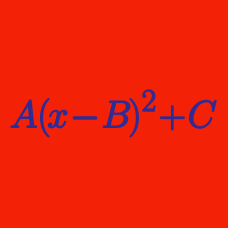Algebra

# Completing the Square - Higher Degree Polynomials

If the function $y=(x^2-2x)^2+16x^2-32x$ has the minimum value $b$ at $x=a$, what is $a-b$?

For $x>0$, the function $y=-x^4+18x^2-74$ has a maximum value $y=b$ at $x=a$. What is $a+b$?

What is the sum of the maximum and minimum values of $y=(x^2-2x+8)^2-16(x^2-2x+8)+84$ in the domain $0 \leq x \leq 3$?

For $x>0$, the function $y=x^4-10x^2+31$ has a minimum value $y=b$ at $x=\sqrt{a}$. What is $a+b$?

For $x>0$, the function $y=-x^4+8x^2-4$ has a maximum value $y=b$ at $x=a$. What is $a+b$?

×